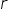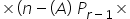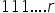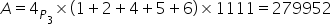Maths-
General
Easy

Question

# Assertion: The sum of all4 digited numbers that can be formed using the digits 1,2,4,5,6 without repetition is 479952Reason: Sum of all‐digit numbers formed by taking the given n digits (without zero) is (sum of all the n digits)(times).

## The correct answer is: A is true, R is true and R is the correct explanation for A

####### With Turito Foundation.#### Get an Expert Advice From Turito.